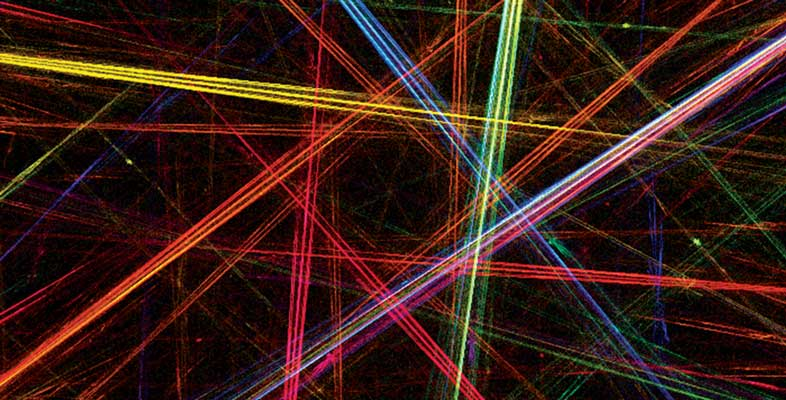Introduction to differentiation

Start this free course now. Just create an account and sign in. Enrol on the course to track your learning.

Free course

# Learning outcomes

After studying this course, you should be able to:

• understand the idea of differentiation from first principles

• differentiate power functions

• find stationary points of some functions

• determine the nature of some stationary points using either the first or second derivative tests

• understand and work with derivatives as rates of change in mathematical models.

MST124_1